# Dear Family, your Operation Maths guide to Number Theory

## Dear Family, your Operation Maths guide to Number Theory

Category : Uncategorized

Dear Family, below is a brief guide to understanding the topic of number theory (fifth and sixth classes only), as well as some practical suggestions as to how you might support your children’s understanding at home. Also below, are a series of links to digital resources that will help both the children, and you, learn more about number theory.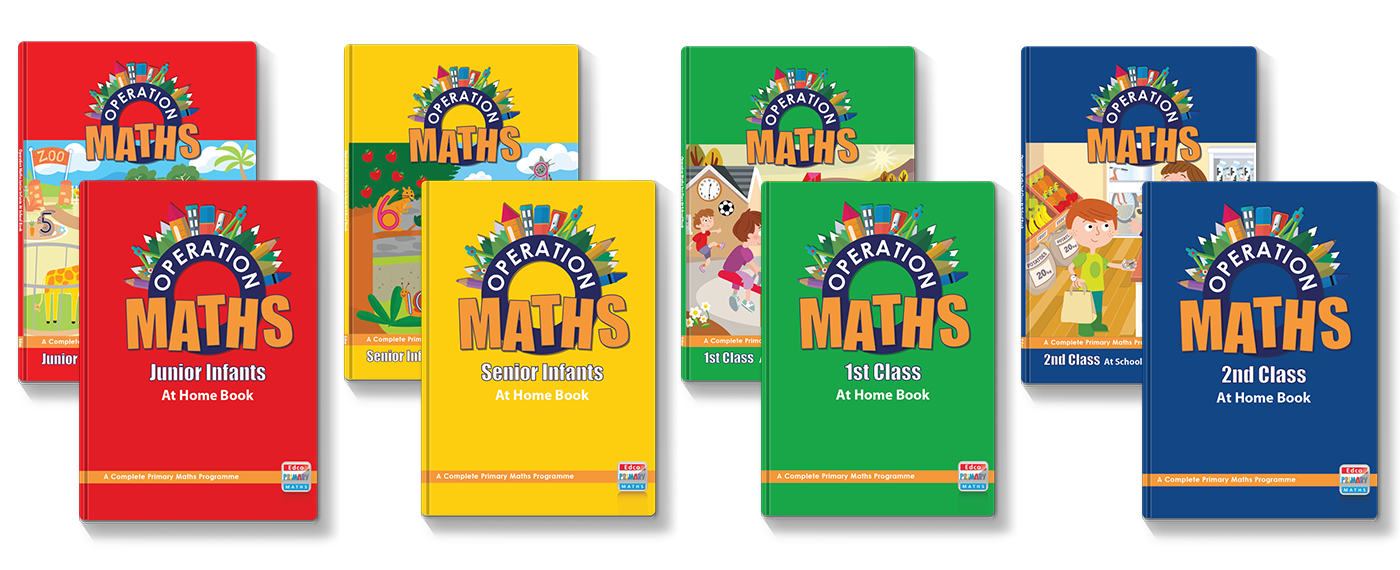You can also find class specific tips in the Operation Maths Dear Family letters for fifth and sixth class.

#### Understanding Number Theory

Number Theory in primary school, is about exploring the shapes of numbers, and how numbers can be visually arranged and represented. It is largely concerned with subcategories of whole numbers:

• odd and even numbers, and what is the resulting number when you add or multiply odd and/or even numbers
• factors and multiples, and how to identify all the possible factor pairs e.g. if you lay out 12 playing cards on a table what are the different ways to arrange them to make a rectangular shape? 1 row of 12, 2 rows of 6, 3 rows of 4 etc
• prime and composite numbers; prime numbers have only one factor pair, the prime number x 1 eg 13 playing cards can only be arranged as 1 row of 13 or 13 rows of 1, which is the same factor pair (1, 13)
• square numbers, triangular numbers and rectangular numbers; some numbers can take the shape of a square (eg 4, 9, 16, 25 etc) and some can take the shape of a triangle (1, 3, 6, 10, 15, 21 etc)
• square roots and exponential numbers.

While Operation Maths 5, for fifth class, is the first book to have a chapter devoted to this topic, the children will have explored elements of this topic in previous classes:

• odd and even numbers were explored formally in Operation Maths 1, 2 and 3
• the language of factors and multiples is used in Operation Maths 3, 4, 5 and 6 as part of the multiplication and division chapters.

The emphasis in Operation Maths is on the child being able to build, manipulate and represent numbers in ways and contexts that are relatable. This will help the child to be better able to visualise numbers, manipulate numbers and understand the connections between them.

#### Practical Suggestions for all Children

• Draw your child’s attention to these number types wherever they or you encounter examples of them.
• Use materials around your home to help make these concepts more real to your child(ren):
• Use pairs of socks, shoes, gloves etc to model addition, subtraction, multiplication and division with odd and even numbers.
• Lay out playing cards, coins, poker chips, plastic cups/plates, lego pieces, toy building blocks etc to represent certain numbers and to explore the shapes that can be made .
• Examine square tiles and/or paving slabs that maybe around your home, how many of these squares together are needed to make a bigger square shape? Are there any patterns? Can you show/build/calculate the first 10 square or triangular numbers?

#### Digital Resources for Fifth and Sixth Classes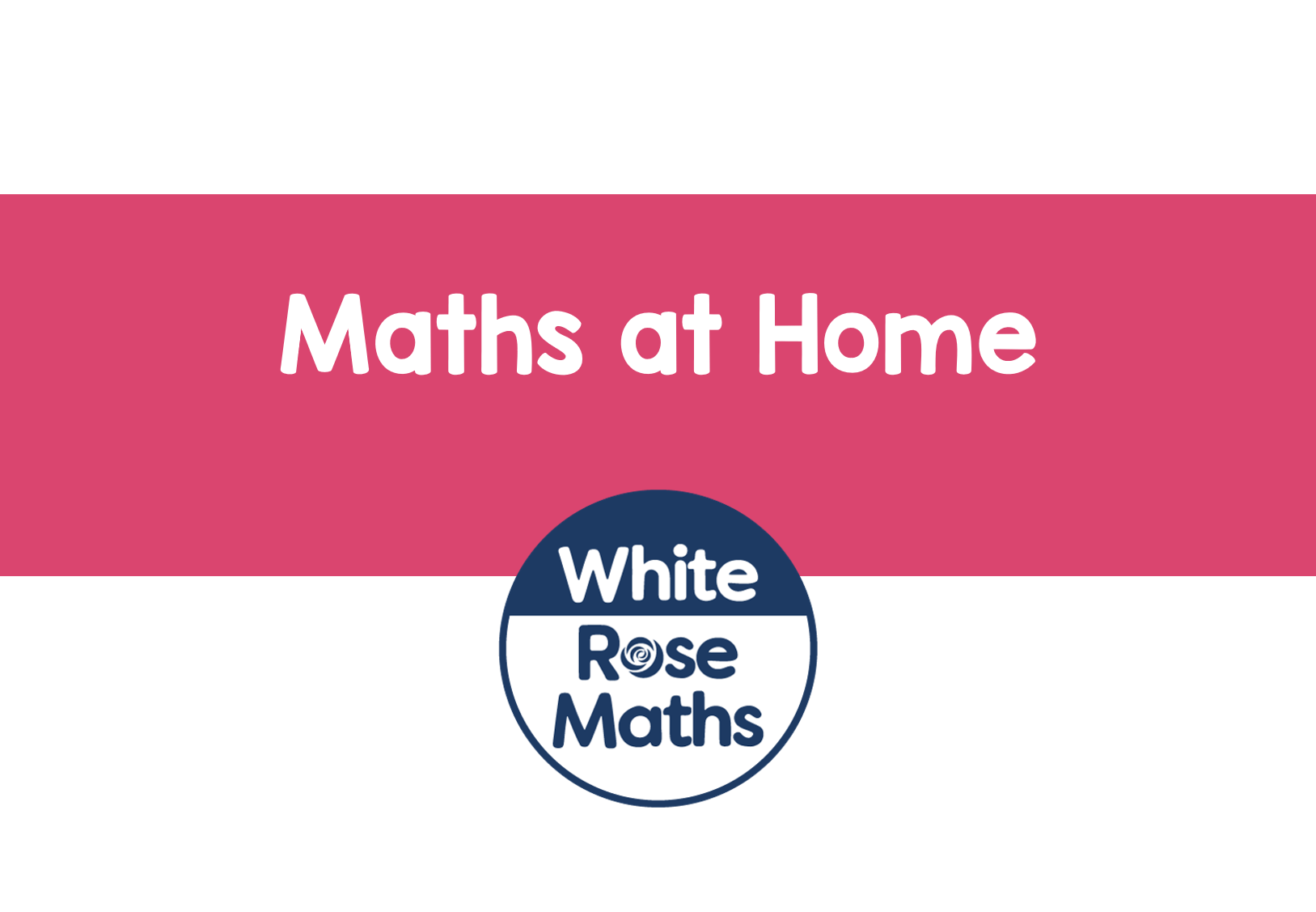White Rose Maths: a series of lessons for Year 5 covering Multiples, Factors and Primes, followed by Square and Cube Numbers. For Year 6 there are lessons that include Factors & Common Factors and Multiples, Primes, Square and Cube Numbers.Matholia: A number of video lessons that include An Introduction to Multiples and An Introduction to FactorsMashup Math – Square Numbers and Square Roots: A video lesson that introduces this concept.Khan Academy: A series of videos and practice questions exploring Odd & Even Numbers (Grade 3), Factors, Multiples, Prime and Composite Numbers (Grade 4) and Intro to Exponents (Grade 6). You can also register for a free Khan Academy account to record your progress and explore other topics/grades.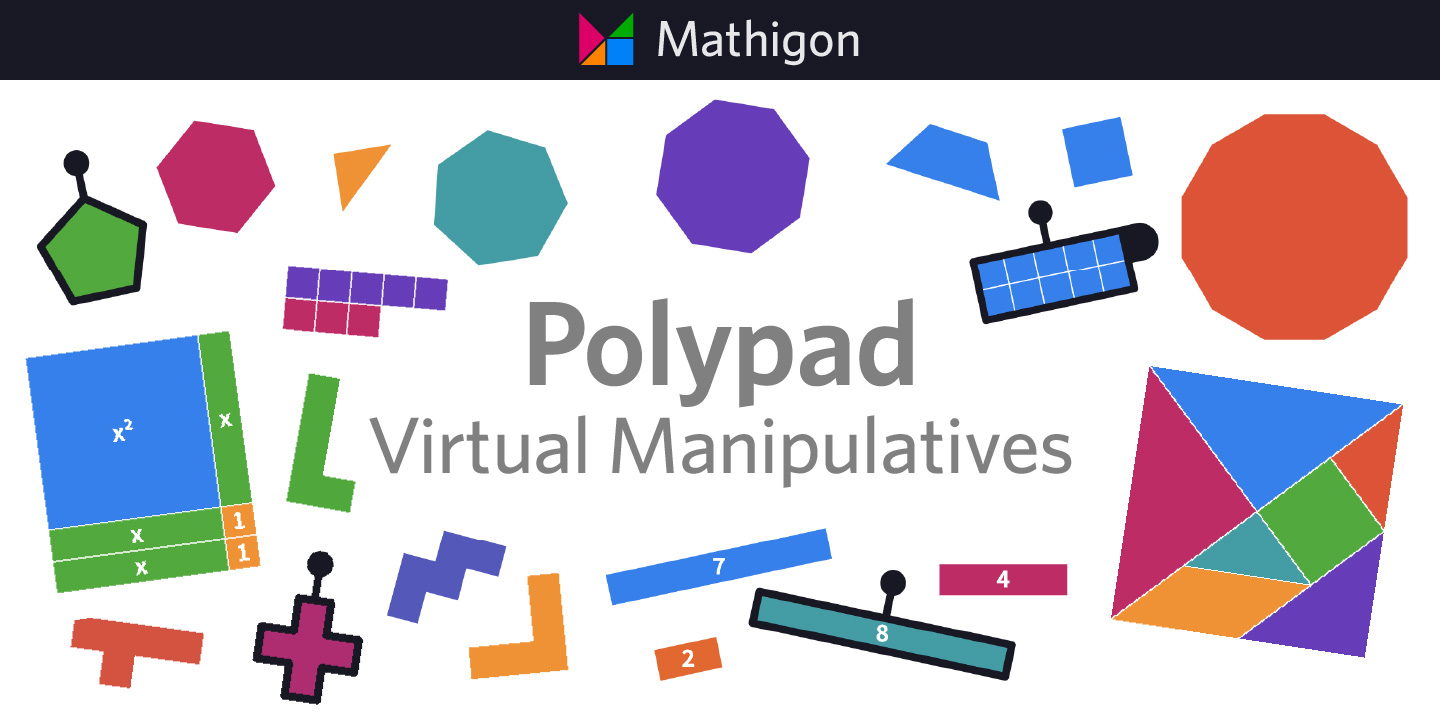Mathigon Polypad: Use this excellent tool to explore and identify factors of any number, and therefore identify if the number is prime or composite. Can also be used to explore square numbers. Select Tiles > Numbers > Number Tiles and drag out tiles to represent a number. Watch here for a video guide (pupils in 5th and 6th class should start with numbers less than 20 first, and then explore bigger numbers when ready)Odd & Even: A selection of games to identify the odd and even numbers; these might serve useful as revision: Coconut Odd or Even, Fruit Splat Odd or Even, Carroll Diagrams Odd and Even.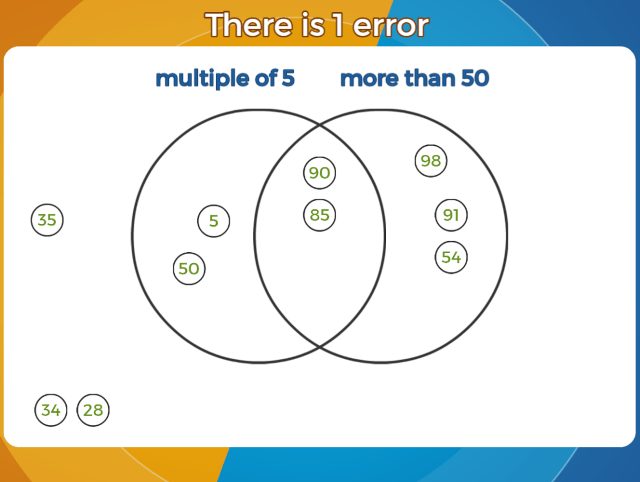Maths Frame – Errors on a Venn Diagram: Identify the numbers which have been placed in the wrong position on the Venn diagram and drag them to their correct place. Choose one or two criteria: to arrange numbers by whether they are odd or even, or multiples of a given number, or prime or square numbers.Multiples and Factors: multiple choice game, finding the Lowest Common Multiple (LCM) or the Highest Common Factor (HCF). Questions increase in difficulty depending on accuracy.Maths Frame – Maths Invaders: Shoot the spaceship with the correct answer and dodge the incoming fire. Select play game and then scroll down to select Recognising Multiples, Factors, Prime, Square and Cube Numbers.PhET – Factors: Choose the Factors option to identify the factors of given numbersSieve of Eratosthene: Use this interactive sieve to remove multiples, and therefore, identify all the prime numbers from 1-400Transum – Factor Trees: Complete the Factor Trees to identify the prime factors. Other related activities can be chosen from the Tabs at the top, including Sieve of Eratosthenes, Pick the Primes, HCF and LCMCubes: use this virtual tool to create models of various cubed numbers; click on the settings cog to input your own dimensions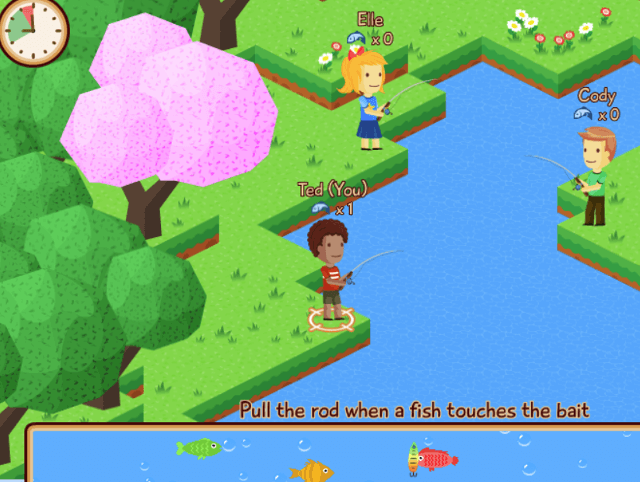Maths Fishing – Square and Cube Numbers: Practise your multiplication skills. Choose game 18, squared numbers, or 19, cubed numbers. A similar game to this is Archery Arithmetic; again, choose game 18 and/or 19That Quiz: Use the factors quiz to practice identifying prime and composite numbers, prime factors, HCF and LCM. Use the exponents quiz to practice roots and exponents.Splash Learn – Algebra Games: These games include Factors, Multiples, Prime and Composite NumbersNumber Theory and Exponents: two sections of practice games from ixl.com. You can do a number of free quizzes each day without having a subscription. Start with the games just below, or at, your class level.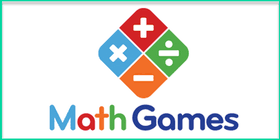Math Games: Practice games on Factors and Multiples, Prime and Composite Numbers, Square Roots. Choose your class level.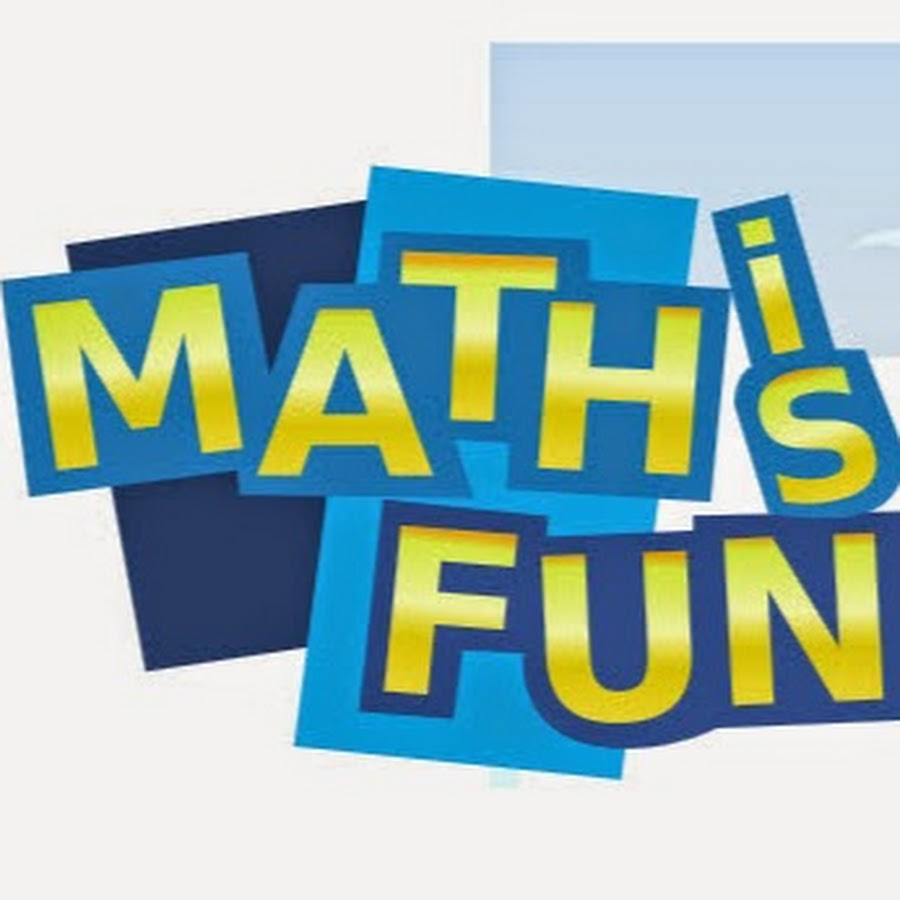Math is Fun: Background information on even and odd numbers, factors and multiples, prime and composite numberssquare numbers and square roots and exponents